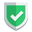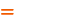我们相信：世界是美好的，你是我也是。平行空间的世界里面，不同版本的生活也在继续...

`pivot`，n.枢轴; 中心; 支点; 核心; 中心点; 最重要的人(或事物);v. (使)在枢轴上旋转(或转动);

## 函数说明

`pivot``Pandas`中的一个函数，可以将一个数据框中的列进行转换，使得某一列成为新的行索引，并用另一列的值来填充这个索引对应的单元格。有如下几个参数：

• `index`: 将要成为新的行索引的列名
• `columns`: 将要成为新的列索引的列名
• `values`: 将要填充新行索引和新列索引之间的单元格的列名

## 标的数据

``````import pandas as pd
df = pd.DataFrame(
{
"name": ["虎子", "老许", "小黑"],
"body": ["A", "B", "C"],
"foot": ["a", "b", "c"]
},
index=["a1", "a2", "a3"],
)
print(df)``````

``````    name   head  body  foot
a1   虎子    10    A    a
a2   老许    30    B    b
a3   小黑    50    C    c``````

## 情况一，参数非数组

``````df_pivot = df.pivot(index='name', columns='head')
print(df_pivot)``````

``````       body           foot
head   10   30   50   10   30   50
name

``````df_pivot = df.pivot(index='name', columns='head', values='body')
print(df_pivot)``````

``````head    10   30   50
name

``````df_pivot = df.pivot(index='name', columns='head')['body']
print(df_pivot,type(df_pivot))``````

``````head   10   30   50
name

<class 'pandas.core.frame.DataFrame'>``````

## 情况二，参数为数组

``````df_pivot = df.pivot(index=['name','head'], columns='body', values='foot')
print(df_pivot)``````

``````body         A    B    C

``````df_pivot = df.pivot(index=['name'], columns=['head','body'], values='foot')
print(df_pivot)``````

``````head   10   30   50
body    A    B    C
name

``````df_pivot = df.pivot(index=["name"], columns=["head"], values=["body", "foot"])
print(df_pivot)``````

``````       body           foot
head   10   30   50   10   30   50
name

## 结束语如果本文对您有帮助，或者节约了您的时间，欢迎打赏瓶饮料，建立下友谊关系。本博客不欢迎：各种镜像采集行为。请尊重原创文章内容，转载请保留作者链接。【福利】 腾讯云最新爆款活动！1核2G云服务器首年50元！【源码】本文代码片段及相关软件，请点此获取更多信息【绝密】秘籍文章入口，仅传授于有缘之人python